# Resources tagged with: Networks/Graph Theory

Filter by: Content type:
Age range:
Challenge level:

### There are 34 results

Broad Topics > Decision Mathematics and Combinatorics > Networks/Graph Theory### Magic W Wrap Up

##### Age 16 to 18Challenge Level

Prove that you cannot form a Magic W with a total of 12 or less or with a with a total of 18 or more.### Geometry and Gravity 2

##### Age 11 to 18

This is the second of two articles and discusses problems relating to the curvature of space, shortest distances on surfaces, triangulations of surfaces and representation by graphs.### Sprouts Explained

##### Age 7 to 18

This article invites you to get familiar with a strategic game called "sprouts". The game is simple enough for younger children to understand, and has also provided experienced mathematicians with. . . .### Network Trees

##### Age 14 to 18Challenge Level

Explore some of the different types of network, and prove a result about network trees.### Some Circuits in Graph or Network Theory

##### Age 14 to 18

Eulerian and Hamiltonian circuits are defined with some simple examples and a couple of puzzles to illustrate Hamiltonian circuits.### Cube Net

##### Age 16 to 18Challenge Level

How many tours visit each vertex of a cube once and only once? How many return to the starting point?### Euler's Formula and Topology

##### Age 16 to 18

Here is a proof of Euler's formula in the plane and on a sphere together with projects to explore cases of the formula for a polygon with holes, for the torus and other solids with holes and the. . . .### Fermat's Poser

##### Age 14 to 16Challenge Level

Find the point whose sum of distances from the vertices (corners) of a given triangle is a minimum.### Magic W

##### Age 14 to 16Challenge Level

Find all the ways of placing the numbers 1 to 9 on a W shape, with 3 numbers on each leg, so that each set of 3 numbers has the same total.### Knight Defeated

##### Age 14 to 16Challenge Level

The knight's move on a chess board is 2 steps in one direction and one step in the other direction. Prove that a knight cannot visit every square on the board once and only (a tour) on a 2 by n board. . . .### Classifying Solids Using Angle Deficiency

##### Age 11 to 16Challenge Level

Toni Beardon has chosen this article introducing a rich area for practical exploration and discovery in 3D geometry### The Bridges of Konigsberg

##### Age 11 to 18Challenge Level

Investigate how networks can be used to solve a problem for the 18th Century inhabitants of Konigsberg.### Instant Insanity

##### Age 11 to 18Challenge Level

Given the nets of 4 cubes with the faces coloured in 4 colours, build a tower so that on each vertical wall no colour is repeated, that is all 4 colours appear.### Tangles

##### Age 11 to 16

A personal investigation of Conway's Rational Tangles. What were the interesting questions that needed to be asked, and where did they lead?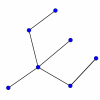### Simply Graphs

##### Age 16 to 18Challenge Level

Look for the common features in these graphs. Which graphs belong together?### Factors and Multiples Graphs

##### Age 16 to 18Challenge Level

Explore creating 'factors and multiples' graphs such that no lines joining the numbers cross### Magic Caterpillars

##### Age 14 to 18Challenge Level

Label the joints and legs of these graph theory caterpillars so that the vertex sums are all equal.### W Mates

##### Age 16 to 18Challenge Level

Show there are exactly 12 magic labellings of the Magic W using the numbers 1 to 9. Prove that for every labelling with a magic total T there is a corresponding labelling with a magic total 30-T.### Olympic Magic

##### Age 14 to 16Challenge Level

in how many ways can you place the numbers 1, 2, 3 … 9 in the nine regions of the Olympic Emblem (5 overlapping circles) so that the amount in each ring is the same?### Plum Tree

##### Age 14 to 18Challenge Level

Label this plum tree graph to make it totally magic!### Symmetric Tangles

##### Age 14 to 16

The tangles created by the twists and turns of the Conway rope trick are surprisingly symmetrical. Here's why!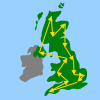### The Olympic Torch Tour

##### Age 14 to 16Challenge Level

Imagine you had to plan the tour for the Olympic Torch. Is there an efficient way of choosing the shortest possible route?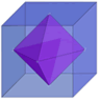### The Four Colour Theorem

##### Age 11 to 16

The Four Colour Conjecture was first stated just over 150 years ago, and finally proved conclusively in 1976. It is an outstanding example of how old ideas can be combined with new discoveries. prove. . . .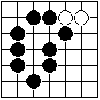### Behind the Rules of Go

##### Age 14 to 18

This article explains the use of the idea of connectedness in networks, in two different ways, to bring into focus the basics of the game of Go, namely capture and territory.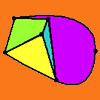### Euler's Formula

##### Age 16 to 18

Some simple ideas about graph theory with a discussion of a proof of Euler's formula relating the numbers of vertces, edges and faces of a graph.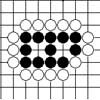### Sufficient but Not Necessary: Two Eyes and Seki in Go

##### Age 14 to 18

The game of go has a simple mechanism. This discussion of the principle of two eyes in go has shown that the game does not depend on equally clear-cut concepts.### Ding Dong Bell

##### Age 11 to 18

The reader is invited to investigate changes (or permutations) in the ringing of church bells, illustrated by braid diagrams showing the order in which the bells are rung.### A Curious Collection of Bridges

##### Age 11 to 18

Read about the problem that tickled Euler's curiosity and led to a new branch of mathematics!### Maximum Flow

##### Age 16 to 18Challenge Level

Given the graph of a supply network and the maximum capacity for flow in each section find the maximum flow across the network.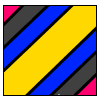### Torus Patterns

##### Age 16 to 18Challenge Level

How many different colours would be needed to colour these different patterns on a torus?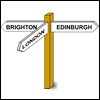### Limiting Probabilities

##### Age 16 to 18Challenge Level

Given probabilities of taking paths in a graph from each node, use matrix multiplication to find the probability of going from one vertex to another in 2 stages, or 3, or 4 or even 100.### An Introduction to Computer Programming and Mathematics

##### Age 16 to 18

This article explains the concepts involved in scientific mathematical computing. It will be very useful and interesting to anyone interested in computer programming or mathematics.### The Use of Mathematics in Computer Games

##### Age 16 to 18

An account of how mathematics is used in computer games including geometry, vectors, transformations, 3D graphics, graph theory and simulations.### Neural Nets

##### Age 16 to 18

Find out some of the mathematics behind neural networks.